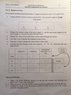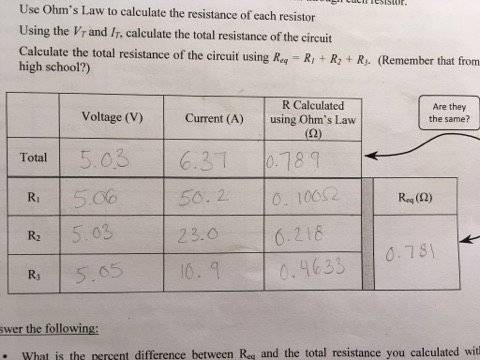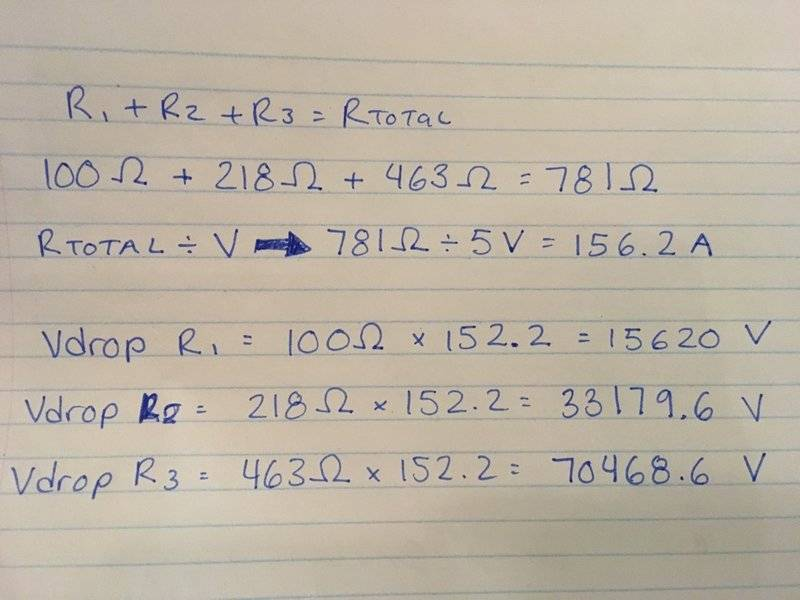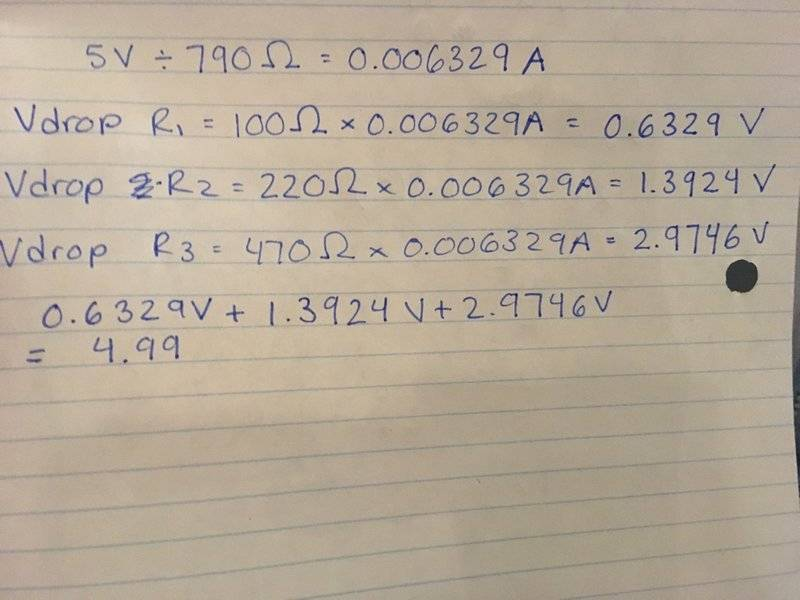# Resistors in Series - Lab data confusion

• shmoop

#### shmoop

Hi guys!

(see my attached photo to better understand where I am coming from!)

So after some research, I've discovered that the current at different points in a simple series circuit is supposed to be the same value, and that the voltage is supposed to be different values.

I performed a lab on resistors in series the other day, and I believe my data is incorrect.
The majority of the data I got tells me that I may have set my circuit up as a parallel series - however the fact that the total measured current is not equal to the sum of the separate currents measured at each resistors conflicts with this. (see attached image of my lab data for clarity!)

Furthermore, due to the value of the total current as well as the value of the total voltage, the total R calculated using Ohm's law is equal to the Req that was calculated through adding the individual resistances up (which were also calculated through Ohm's law).

I am wondering, what did I do to obtain this kind of data information? As it does not conform what I would designate as a series circuit, nor a parallel circuit.

Does anyone have any idea what I have done to obtain this lab data? What kind of circuit did I create?

Edit: Here are the resistor values:

R1=100 ohms
R2= 220 ohms
R3= 470 ohms

#### Attachments

•21362985_10212281109076164_594219238_n (1).jpg
22.2 KB · Views: 441
Last edited by a moderator:

I think you wired it in series but wrote the current values in the voltage column and the voltage values in the current column.

Edit: oh darn that can't be the only problem as I doubt you were playing with 50volts.

•shmoop
I think you wired it in series but wrote the current values in the voltage column and the voltage values in the current column.
I considered that too. But I followed the formula R=V/I, and the values worked out that way. If I divide the current values by the voltage values instead, the resistance doesn't work out like it should...

If I divide the current values by the voltage values instead, the resistance doesn't work out like it should...

you SHOULDNT be dividing current by voltage ... .it will never give you the correct answer

•shmoop
you SHOULDNT be dividing current by voltage ... .it will never give you the correct answer
I know haha! But I was responding to CWatters who had mentioned that it looked like my current and voltage values were reversed. In which case I would do the opposite of what I had previously done, therefore dividing the current by the voltage.

(see my attached photo to better understand where I am coming from!)

What is the battery voltage ?
are the resistance values given ... even at least one of them ?

•shmoop

What is the battery voltage ?
are the resistance values given ... even at least one of them ?

Here is a zoomed in version of the photograph!

The supply was approximately 5 volts.

R1=100 ohms
R2= 220 ohms
R3= 470 ohms

#### Attachments

The majority of the data I got tells me that I may have set my circuit up as a parallel series - however the fact that the total measured current is not equal to the sum of the separate currents measured at each resistors conflicts with this. (see attached image of my lab data for clarity!)

you only need to work out the current through 1 resistor to work out the total current in the circuit
you DONT add it up as you do with the voltage drops across each resistor

•shmoop
you only need to work out the current through 1 resistor to work out the total current in the circuit
you DONT add it up as you do with the voltage drops across each resistor

How would you mathematically determine the total current in the circuit with the current through 1 resistor?
I know that you don't add it up for a series circuit - I was trying to say that that is what I would have done for a parallel circuit. I wrote series accidentally! Sorry. I meant to say the majority of my data makes it look like I set up the situation as a parallel circuit. (all the different current values, and the fact that the voltage stays the same)OK that is easier to read

the figures in your current column cannot be correct
and I don't know why they are asking you to calculate resistor values for column 3 when the values are already given to you ??

•shmoop
View attachment 210432

OK that is easier to read

the figures in your current column cannot be correct
and I don't know why they are asking you to calculate resistor values for column 3 when the values are already given to you ??

I assume they ask so that you can then use the values to perform the Rtotal=1/R1+1/R2+1/R3

To prove that it is a series circuit

How would you mathematically determine the total current in the circuit with the current through 1 resistor?

you treat all series resistors as 1 resistor ... add up those 3 values for a total resistance value

then divide the voltage (5V) by the total resistance to get your current

then you can look at the voltage drops across each resistor

Vdrop R1 = resistance x current =

Vdrop R2 = resistance x current =

Vdrop R3 = resistance x current =

adding up those 3 voltages should equal your supply voltage = 5V

•I assume they ask so that you can then use the values to perform the Rtotal=1/R1+1/R2+1/R3

no, that formula is for parallel resistors

•shmoop
no, that formula is for parallel resistors

Oopsies. I meant to make sure it matches the total R with the Rtotal=R1+R2+R3

•davenn
Oopsies. I meant to make sure it matches the total R with the Rtotal=R1+R2+R3

OK

so copy and paste that post #12 of mine and fill in the answers

•shmoop
you treat all series resistors as 1 resistor ... add up those 3 values for a total resistance value

then divide the voltage (5V) by the total resistance to get your current

then you can look at the voltage drops across each resistor

Vdrop R1 = resistance x current =

Vdrop R2 = resistance x current =

Vdrop R3 = resistance x current =

adding up those 3 voltages should equal your supply voltage = 5V

My voltages are 5V throughout. Which is confusing. Because they equal 15V when summed, as opposed to 5V. However, when calculating my individual resistance values, when summed they equaled the total resistance initially calculated. This is what confuses me

My voltages are 5V throughout.

they cannot be 5V throughout because that is your total voltage

•shmoop
have you copied and pasted this ?

you treat all series resistors as 1 resistor ... add up those 3 values for a total resistance value

then divide the voltage (5V) by the total resistance to get your current

then you can look at the voltage drops across each resistor

Vdrop R1 = resistance x current =

Vdrop R2 = resistance x current =

Vdrop R3 = resistance x current =

adding up those 3 voltages should equal your supply voltage = 5V

just a note I didn't state when I typed that out originally
should be ( for clarity)

Vdrop R1 = resistance R1 x current =

Vdrop R2 = resistance R2 x current =

Vdrop R3 = resistance R3 x current =

•shmoop
have you copied and pasted this ?

you treat all series resistors as 1 resistor ... add up those 3 values for a total resistance value

then divide the voltage (5V) by the total resistance to get your current

then you can look at the voltage drops across each resistor

Vdrop R1 = resistance x current =

Vdrop R2 = resistance x current =

Vdrop R3 = resistance x current =

adding up those 3 voltages should equal your supply voltage = 5V

just a note I didn't state when I typed that out originally
should be ( for clarity)

Vdrop R1 = resistance R1 x current =

Vdrop R2 = resistance R2 x current =

Vdrop R3 = resistance R3 x current =

The values that were measured were the voltage and the current. The resistances were calculated from these. For me to perform these calculations seems aimless, as I will just get ~5V for each voltage drop. Which is part of my main issue.

The values that were measured were the voltage and the current. The resistances were calculated from these. For me to perform these calculations seems aimless, as I will just get ~5V for each voltage drop.

it's not aimless as you will find your mistake
AGAIN, there is NOT a 5V drop across each resistor --- it's impossible to have a 5V drop across each resistor

Copy and Paste ( DONT QUOTE IT) my post and do the sums as I have laid them out and you will see where you have gone wrongDave

•it's not aimless as you will find your mistake
AGAIN, there is NOT a 5V drop across each resistor --- it's impossible to have a 5V drop across each resistor

Copy and Paste ( DONT QUOTE IT) my post and do the sums as I have laid them out and you will see where you have gone wrongDaveI am confused

I am confused

Im not surprised

what is Ohms law ? as you didn't use it correctly to find the current

and since you got the incorrect current, everything following that is incorrect

I also notice you didn't use the resistor values you stated in post 1

100 Ohms 220 Ohms 470 Ohms

you didn't do your working on the post so I couldn't copy and paste individual parts

•shmoop
Im not surprised

what is Ohms law ? as you didn't use it correctly to find the current

and since you got the incorrect current, everything following that is incorrect

I also notice you didn't use the resistor values you stated in post 1

100 Ohms 220 Ohms 470 Ohms

you didn't do your working on the post so I couldn't copy and paste individual parts

Woops. Huge mistake there. I will redo it. I thought I was supposed to use my experimental values.

•davenn
we will get you there, you just need to get the basics correctIf I give you the answers, you won't learn anything, because you still won't understand where you went wrong•shmoop
Woops. Huge mistake there. I will redo it. I thought I was supposed to use my experimental values.

that's OK we can work with the actual values rather than the idea values you originally posted
it's just your answers will be a little different, that's all•shmoop
that's OK we can work with the actual values rather than the idea values you originally posted
it's just your answers will be a little different, that's allI see! So these are the voltage drops!

•davenn
I see! So these are the voltage drops!

YES ! well donenone of the individual drops can = 5V, they all must be smaller
and when added together will = 5V ( or whatever the supply voltage is)

•shmoop
Now you could redo the math using your actual resistor values
you should again end up with 5V ( as a total drop) give or take a couple of decimal points

each individual drop will be a little different to what you just worked out for the ideal resistor values

•shmoop
Now that we have the resistor values I think I can see what you did in the experiment.

It looks to me like you started with the resistors in series and measured the overall current correctly (but forgot the current values were in mA not A).

For example the total resistance is 790 Ohms so the current should be about 6.37mA but you wrote down 6.37A. Then when calculating the total resistance you did R=V/I (which is correct method) but you used:
R = 5.03/6.37 = 0.789 Ohms
R = 5.03/(6.37 * 10-3) = 789 Ohms (very close to 790 Ohms).

Then when you came to measuring the current through each resistor you connected each resistor to the power supply on it's own, which is incorrect. So the voltage across each resistor was the same about 5.03V.

The currents should have been...

For R1... I = 5.03/100 = 50.3mA (You recorded 50.2A)
For R2... I = 5.03/220 = 22.8mA (You recorded 23A)
For R3... I = 5.03/470 = 10.7mA (You recorded 10.9A)

So again it just looks like you forgot the values were in mA.

Again this made all your resistance calculations out by a factor of 100. Your calculations should have given you..

R1 = 100 Ohms
R2 = 218 Ohms
R3 = 463 Ohms

These are all within tolerance.

Of course you should have left all the resistors in series and measured the voltage across each.

•shmoop
How did you calculate the resistance with those current values?

In a series circuit, the current value is supposed to stay the same, and the voltage value is supposed to vary.

How did you calculate the resistance with those current values?

In a series circuit, the current value is supposed to stay the same, and the voltage value is supposed to vary.

That's what got me thinking that you didn't have all three resisters in series when measuring the current through each resistors . If you changed the circuit and connected each resistor on it's own to the power supply (or put them all in parallel) then the voltage would be the same each time and the current values would change. The calculated current values are very close to the figures you measured if you allow for the mA vs A error.

So using your data with the current corrected to mA...

R1 = 5.06/(50.2 * 10-3) = 100.8 Ohms
R2 = 5.03/(23.0 * 10-3) = 218.7 Ohms
R3 = 5.05/(10.9 * 10-3) = 463.3 Ohms

Which are close to the correct values.

•shmoop
That's what got me thinking that you didn't have all three resisters in series when measuring the current through each resistors . If you changed the circuit and connected each resistor on it's own to the power supply (or put them all in parallel) then the voltage would be the same each time and the current values would change. The calculated current values are very close to the figures you measured if you allow for the mA vs A error.

So using your data with the current corrected to mA...

R1 = 5.06/(50.2 * 10-3) = 100.8 Ohms
R2 = 5.03/(23.0 * 10-3) = 218.7 Ohms
R3 = 5.05/(10.9 * 10-3) = 463.3 Ohms

Which are close to the correct values.

Yes, I believe you are very correct. I must have disconnected the series circuit in the process of attempting to measure the voltage drops.

Thanks a lot for your input!

Now you could redo the math using your actual resistor values
you should again end up with 5V ( as a total drop) give or take a couple of decimal points

each individual drop will be a little different to what you just worked out for the ideal resistor values

Thank you very much! You were a great help in having me figure out what my voltage drops were. I appreciate it

•davenn
You can read the resisters by their color codes or you can measure them one at a time, stand alone. That will help greatly. For resisters in series there are three important things to consider that your data has completely wrong:
1) The current through all resisters will be equal.
2) The voltage drop over any resister is proportional to the resistance.
3) The total voltage drop is the sum of the individual voltage drops across the resisters.

You should make sure that your measurements agree with these principles for resisters in series before you precede.

•shmoop